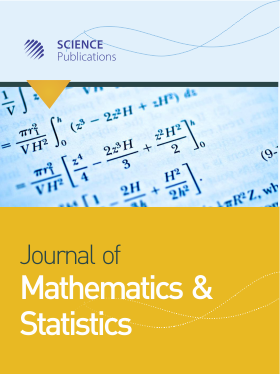Frequency: Continuous
ISSN: 1549-3644 (Print)
ISSN: 1558-6359 (Online)
Research Article Open Access

# Negative Binomial-Lindley Distribution and Its Application

Hossein Zamani1 and Noriszura Ismail1
• 1 ,
Journal of Mathematics and Statistics
Volume 6 No. 1, 2010, 4-9

DOI:

Submitted On: 10 November 2009 Published On: 31 March 2010

How to Cite: Zamani, H. & Ismail, N. (2010). Negative Binomial-Lindley Distribution and Its Application. Journal of Mathematics and Statistics, 6(1), 4-9. https://doi.org/10.3844/jmssp.2010.4.9

## Abstract

Problem statement: The modeling of claims count is one of the most important topics in actuarial theory and practice. Many attempts were implemented in expanding the classes of mixed and compound distributions, especially in the distribution of exponential family, resulting in a better fit on count data. In some cases, it is proven that mixed distributions, in particular mixed Poisson and mixed negative binomial, provided better fit compared to other distributions. Approach: In this study, we introduce a new mixed negative binomial distribution by mixing the distributions of negative binomial (r,p) and Lindley (θ), where the reparameterization of p = exp(-λ) is considered. Results: The closed form and the factorial moment of the new distribution, i.e., the negative binomial-Lindley distribution, are derived. In addition, the parameters estimation for negative binomial-Lindley via the method of moments (MME) and the Maximum Likelihood Estimation (MLE) are provided. Conclusion: The application of negative binomial-Lindley distribution is carried out on two samples of insurance data. Based on the results, it is shown that the negative binomial-Lindley provides a better fit compared to the Poisson and the negative binomial for count data where the probability at zero has a large value.

• 2,247 Views